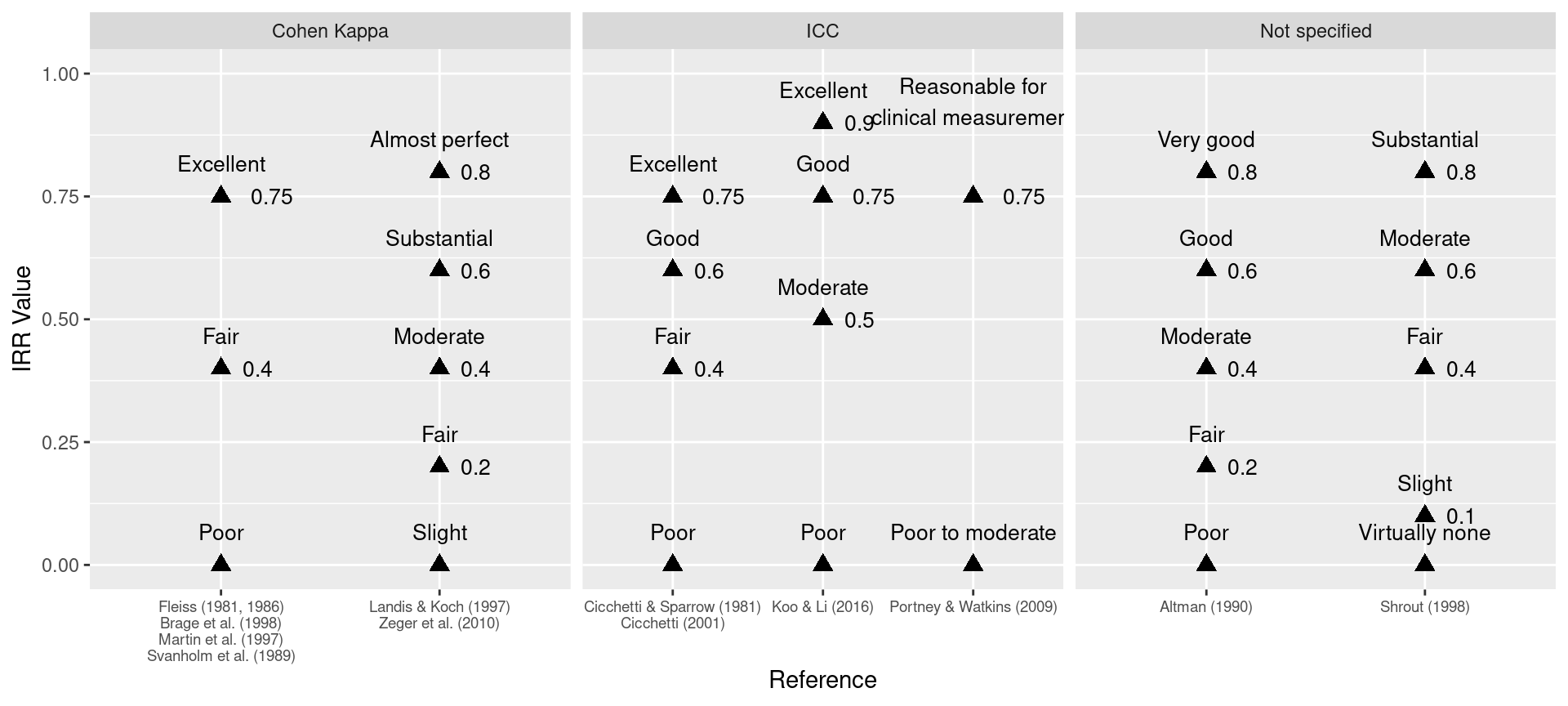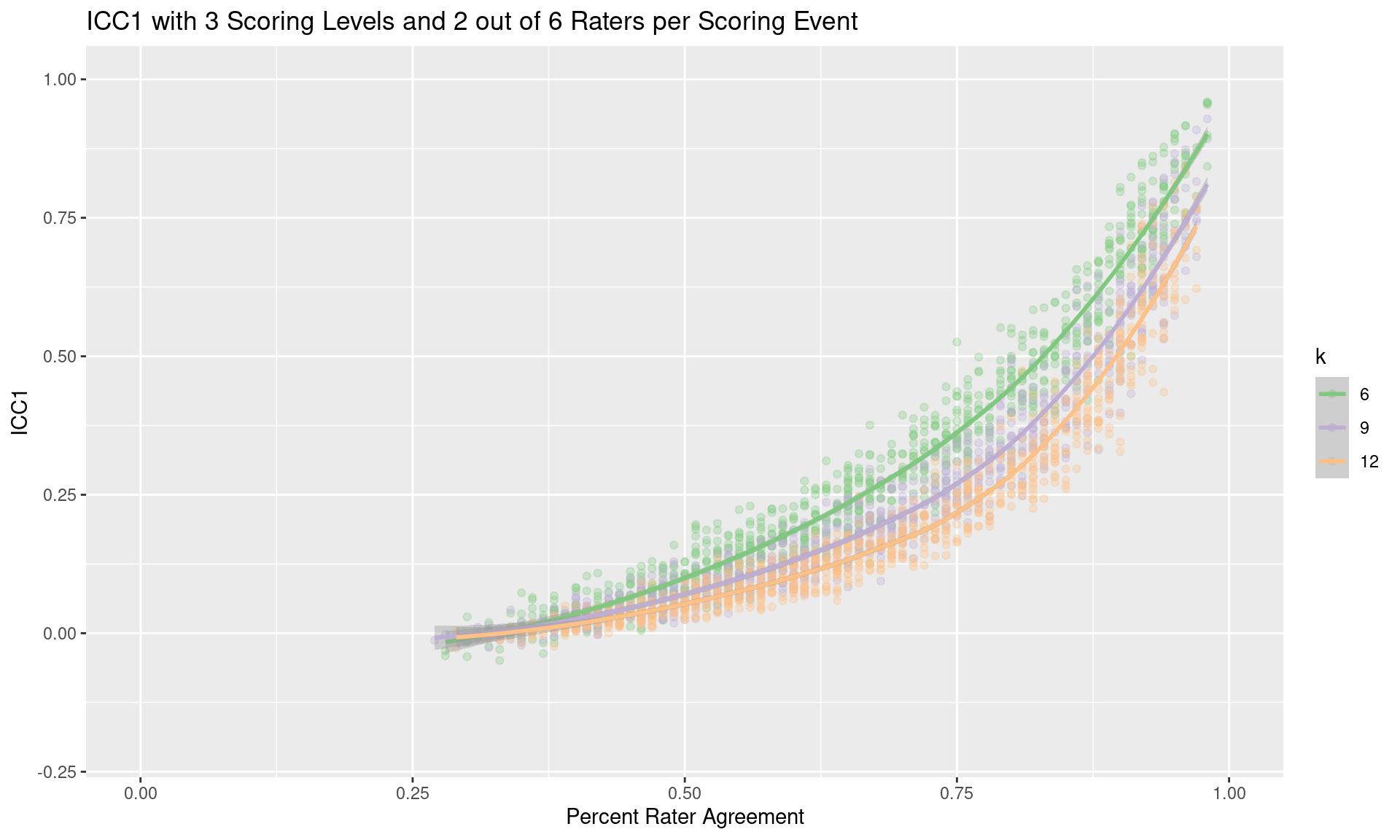April 11, 2023

## Motivation

• The Diagnostic Assessment and Achievement of College Skills (DAACS; www.DAACS.net) is a suite of technological and social supports to optimize student learning. DAACS provides personalized feedback about students’ strengths and weaknesses in terms of key academic (mathematics, reading, & writing) and self-regulated learning skills, linking them to the resources to help them be successful students.
• For the writing assessment, were trying to establish inter-rater reliability in two ways:
1. Human-to-human
2. Human-to-machine
• Literature clearly suggests ICC is the appropriate measure, however most of that research is from the medical literature.
• Guidance on interpretation of ICC is not very clear.

## Guiding Research Questions

1. What is the relationship between intraclass correlation (ICC) and percent rater agreement (PRA)?
2. Are the published guidelines for interpreting ICC appropriate for all rating designs?

## Measuring Inter-rater Reliability

Percent Rater Agreement (PRA)

• Percentage of scoring events where raters’ scores agree.

Intraclass Correlation (ICC)

• ICC is a quantitative measurements are made on units that are organized into groups. It describes how strongly units in the same group resemble each other

Cohen’s Kappa

• Measure of agreement between two raters that takes into account agreement by chance.

Fleiss’ Kappa

• Extension of Cohen’s kappa for more than two raters. It also takes into account agreement by chance.

## Components of Measuring IRR

Models:

• One-way random effects: each subject is measured by a different set of k randomly selected raters.
• Two-way random effects: k raters are randomly selected, then, each subject is measured by the same set of k raters.
• Two-way mixed effects: k fixed raters are defined. Each subject is measured by the k raters.

Number of measurements:

• Single measures: even though more than one measure is taken in the experiment, reliability is applied to a context where a single measure of a single rater will be performed.
• Average measures: the reliability is applied to a context where measures of k raters will be averaged for each subject.

Consistency or absolute agreement:

• Absolute agreement: the agreement between two raters is of interest, including systematic errors of both raters and random residual errors.
• Consistency: in the context of repeated measurements by the same rater, systematic errors of the rater are canceled and only the random residual error is kept.
IRR Statistic Description Formula
Percent Agreement One-way random effects; Absolute agreement $$\frac{number\ of\ observations\ agreed\ upon}{total\ number\ of\ observations}$$
ICC(1,1) One-way random effects; absolute agreement; single measurements $$\frac{MS_R - MS_W}{MS_R + (k - 1)MS_W}$$
ICC(2,1) Two-way random effects; absolute agreement; single measures $$\frac{MS_R - MS_W}{MS_R + (k - 1)MS_E + \frac{k}{n}(MS_C - MS_E)}$$
ICC(3,1) Two-way random mixed effects; consistency; single measures. $$\frac{MS_R - MS_E}{MS_R + (k-1)MS_E}$$
ICC(1,k) One-way random effects; absolute agreement; average measures. $$\frac{MS_R - MS_W}{MS_R}$$
ICC(2,k) Two-way random effects; absolute agreement; average measures. $$\frac{MS_R - MS_E}{MS_R | \frac{MS_C - MS_E}{n}}$$
ICC(3,k) Two-way mixed effects; consistency; average measures. $$\frac{MS_R - MS_E}{MS_R}$$
Cohen’s Kappa Absolute agreement $$\frac{P_o - P_e}{1 - P_e}$$

Note. $$MS_R$$ = mean square for rows; $$MS_W$$ = mean square for residual sources of variance; $$MS_E$$ = mean square error; $$MS_C$$ = mean square for columns; $$P_o$$ = observed agreement rates; $$P_e$$ = expected agreement rates.

## Guidelines for Interpreting IRR

Altman (1990) (Not specified)
• < 0.2 Poor
• 0.2 - 0.4 Fair
• 0.4 - 0.6 Moderate
• 0.6 - 0.8 Good
• >0.8 Very good
• Cicchetti & Sparrow (1981); Cicchetti (2001) (ICC, Cohen Kappa)
• < 0.4 Poor
• 0.4 - 0.6 Fair
• 0.6 - 0.75 Good
• >0.75 Excellent
• Fleiss (1981, 1986); Brage et al. (1998); Martin et al. (1997); Svanholm et al. (1989) (Cohen Kappa)
• < 0.4 Poor
• 0.4 - 0.75 Fair
• >0.75 Excellent
• Koo & Li (2016) (ICC)
• < 0.5 Poor
• 0.5 - 0.75 Moderate
• 0.75 - 0.9 Good
• >0.9 Excellent
• Landis & Koch (1997); Zeger et al. (2010) (Cohen Kappa)
• < 0.2 Slight
• 0.2 - 0.4 Fair
• 0.4 - 0.6 Moderate
• 0.6 - 0.8 Substantial
• >0.8 Almost perfect
• Portney & Watkins (2009) (ICC)
• < 0.75 Poor to moderate
• >0.75 Reasonable for clinical measurement
• Shrout (1998) (Not specified)
• < 0.1 Virtually none
• 0.1 - 0.4 Slight
• 0.4 - 0.6 Fair
• 0.6 - 0.8 Moderate
• >0.8 Substantial
• ## Guidelines for Interpreting IRR## Guidelines for Education

In education, ICC(1,1) is often the most appropriate intraclass correlation (ICC) metric to use given each subject is measured by two randomly selected raters (one-way), single measures are used, and absolute agreement is desired (Shrout & Fleiss, 1979).

Guidelines for interpreting ICC is largely found in the medical literature. When discussing to ICC(1,1), Koo and Li (2016) state that “practically, this model is rarely used in clinical reliability analysis because majority of the reliability studies typically involve the same set of raters to measure all subjects” (pp. 156-157).

The guidelines for interpreting ICC may not be appropriate for all forms of ICC. As will be shown, the magnitude of the ICC vary greatly for the same percent agreement.

## The IRRsim R Package

The IRRsim package provides functions to simulate various scoring designs.

devtools::install_github('jbryer/IRRsim')
library(IRRsim)

Key functions:

• simulateRatingMatrix - Simulate a single rating matrix.
• simulateIRR - Simulates many scoring matrices with varying percent rater agreements. S3 methods implemented for objects returned by simulateIRR:
• summary
• plot
• as.data.frame
• IRRsim_demo - Run an interactive Shiny application.

## simulateRatingMatrix

Simulate a single rating matrix.

For each scoring event (i.e. row within a scoring matrix):

1. One of k raters is randomly selected.
2. A score, y, is randomly selected from the response distribution (uniform distribution by default)
3. A random number, x, between 0 and 1 is generated.
• If x is less than the specified desired percent agreement, the remaining values in the row are set to y.
• Otherwise, scores for the remaining raters are randomly selected from the response distribution.
4. Repeat steps 1 through 3 for the remaining scoring events.
5. If $$k_m < k$$, then $$k - k_m$$ scores per row are set to NA (missing).

## Simulating Scoring Matrices

set.seed(2112)
test1 <- simulateRatingMatrix(
nLevels = 3, k = 6, k_per_event = 6,
agree = 0.6, nEvents = 10)
test1
##    aa ab ac ad ae af
## 1   1  1  1  1  1  1
## 2   2  2  2  2  2  2
## 3   1  1  1  1  1  1
## 4   1  1  1  2  1  1
## 5   2  1  2  1  3  1
## 6   2  2  2  2  2  2
## 7   2  2  2  2  2  2
## 8   2  2  1  1  1  2
## 9   2  2  2  2  2  2
## 10  3  1  3  2  3  2
agreement(test1)
##  0.6
set.seed(2112)
test2 <- simulateRatingMatrix(
nLevels = 3, k = 6, k_per_event = 2,
agree = 0.6, nEvents = 10)
test2
##    aa ab ac ad ae af
## 1  NA  1 NA  1 NA NA
## 2  NA NA NA NA  2  2
## 3   1 NA NA NA  1 NA
## 4  NA NA  1 NA  1 NA
## 5  NA NA  2 NA NA  1
## 6   2 NA  2 NA NA NA
## 7  NA  2 NA  2 NA NA
## 8  NA NA  1 NA NA  2
## 9  NA NA NA  2  2 NA
## 10  3  1 NA NA NA NA
agreement(test2)
##  0.7

## simulateIRR

Simulates many scoring matrices with varying percent rater agreements. For each scoring matrix, IRR statistics are calculated. This functions returns an object of type IRRsim which has S3 methods defined for plot, summary, and as.data.frame.

For the remainder of the document, we wish to estimate ICC for 6, 9, and 12 raters under the conditions of 3, 5, and 9 scoring levels.

tests.3levels <- simulateIRR(nRaters = c(6, 9, 12), nRatersPerEvent = 2, nLevels = 3)
tests.5levels <- simulateIRR(nRaters = c(6, 9, 12), nRatersPerEvent = 2, nLevels = 5)
tests.9levels <- simulateIRR(nRaters = c(6, 9, 12), nRatersPerEvent = 2, nLevels = 9)

Note that if the parameter parallel = TRUE is specified, the function will use multiple cores if available.